Rating : ⭐⭐⭐⭐⭐
Price : \$10.99
Language:EN
Pages: 2

# Find the thvenin equivalent this circuit over what portions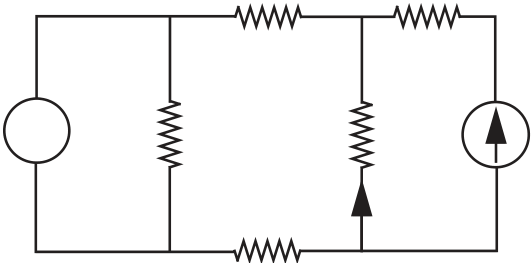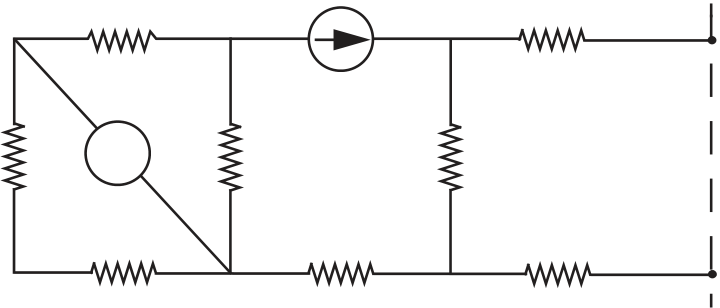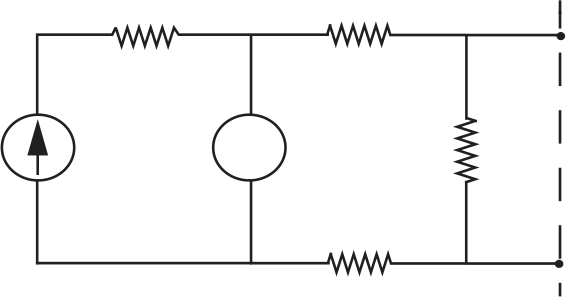182 C H A P T E R

T H R E E

e x e r c i s e 3.21
a) Write in standard form the minimum number of node equations needed to analyze the circuit in Figure 3.122.

FIGURE 3.122

v
R1 R2 i4 R4
R3

e x e r c i s e 3.22

FIGURE 3.123

a) Find the Thévenin equivalent of the circuit in Figure 3.123.
R3 I R7
R1 +-

FIGURE 3.124

b) R2 R5 R8

A

R1 R2 1
I
R4
R3 1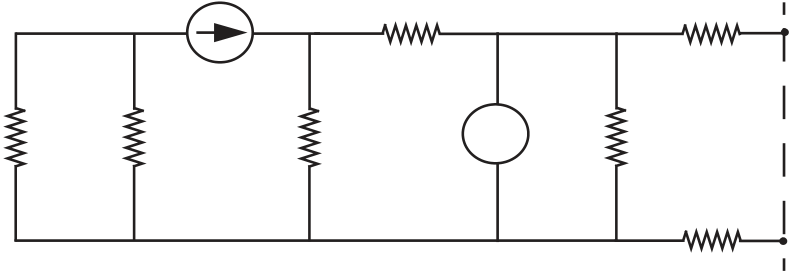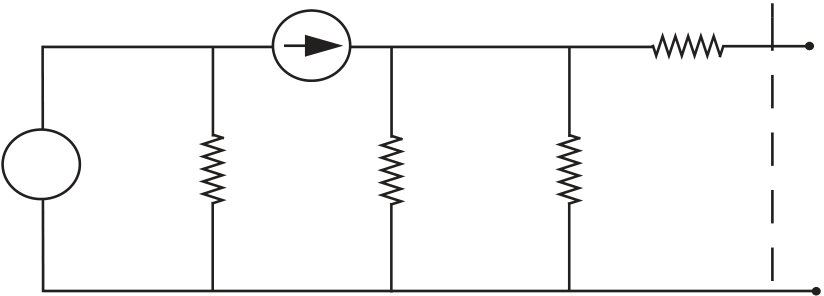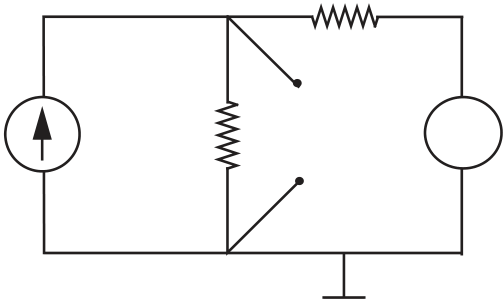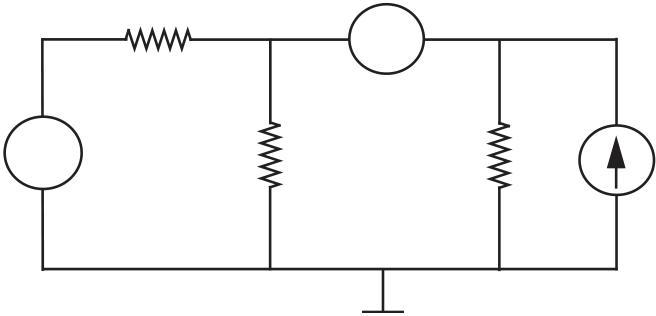b) I R4

R6

1
183
R1 R2 R3 v +
-

R7

1

FIGURE 3.125

A
I R4
v

R2

R3

A

e x e r c i s e 3.25
a 2 V

-
+

FIGURE 3.127

b
5 V +
-
8kΩ

+ -

8kΩ 0.4kΩ 2.5mA
e x e r c i s e 3.26 For the circuit in Figure 3.129, write the node equations. Do

not solve, but write in matrix form: source terms on the left, unknown variables on the right.

How It Works